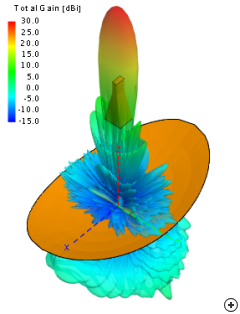# Antenna Arrays And Python - Calculating Directivity

Directivity is a measure of how directional an antenna’s radiation pattern is. For example an antenna that radiates strongly in one direction has a high directivity while an antenna that radiates equally in all directions has a low directivity.

It is not necessarily a bad thing to have an antenna with low directivity, it depends on the application. For example:

To communicate with a geosynchronous satellite 35,786km away we need an antenna that produces a strong beam directed towards the satellite. In this case a parabolic dish antenna is often used because it has a high directivity.

Alternatively a mobile phone needs to be able to connect to a cell tower no matter what orientation the phone is held which means no matter what direction it’s antenna is pointing. In this case an antenna with a low directivity is used so that the antenna can receive/transmit well in any direction.Mobile Phone Pattern — Low Directivity, Dish Pattern — High Directivity

Calculating Directivity

Directivity can be calculated using the equation below which basically means the max value of radiated power divided by the average power in all directions:

Where:

Directivity is often expressed in dBi and represents the dB ratio with respect to an isotropic radiator.

Python Script - Directivity.py

The gist below shows my Python script for calculating the directivity (based on the ArrayCalc calc_directivity.m file).

The main function is:

`CalcDirectivity(Efficiency, RadPatternFunction, *args)`

This takes a function argument, RadPatternFunction, as an input. This function should describe the antennas radiation pattern in terms of theta and phi.

At the bottom of the script there are some basic examples showing how to calculate the directivity for three different radiation patterns - an isotropic antenna and two sin functions. Below this there are also two examples calculating the directivity for rectangular patches using the functions discussed in my Square Patch Element post.

The efficiency argument to RadPatternFunction is related to the Gain of the antenna and will be discussed in my next post.